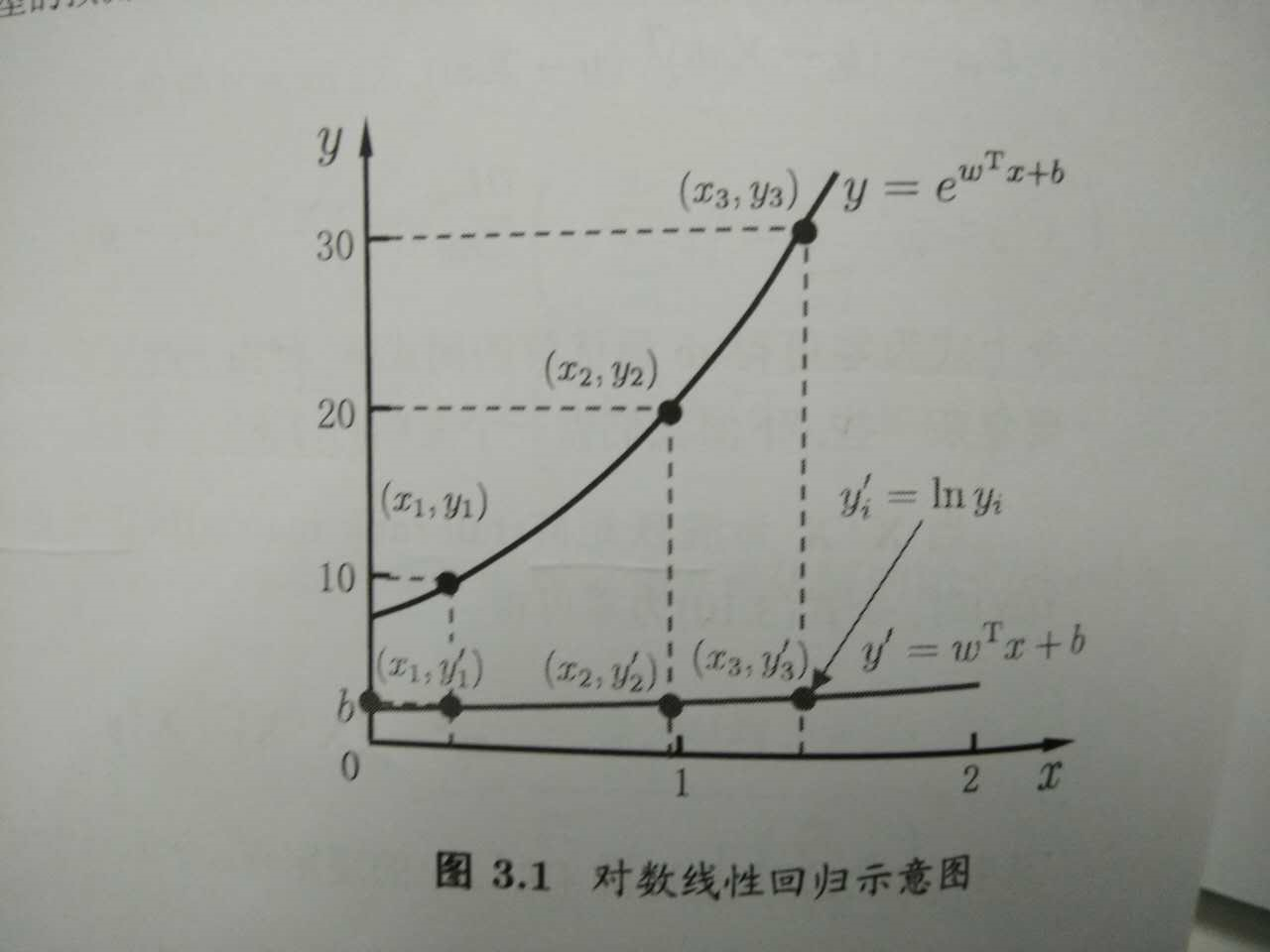• 对数线性回归
2022-06-21 17:03:45

# R语言使用glm函数构建泊松对数线性回归模型处理三维列联表数据构建饱和模型、使用summary函数获取模型汇总统计信息

目录r语言 数据挖掘 人工智能 机器学习
更多相关内容
• 对数线性回归； y的衍生物 3.1 基本形式 假设样本x有d个属性，线性模型（linear model）试图学得一个通过属性的线性组合来进行预测的函数，即f(x)=w1x1+w2x2+⋅⋯+wdxd+bf(x)=w1x1+w2x2+⋅⋯+wdxd+bf(x)=w_{1}x_...

墙裂推荐阅读：ｙ的衍生物

关键词：最小二乘法；正则化；对数线性回归； y的衍生物

## 3.1 基本形式

假设样本x有d个属性，线性模型（linear model）试图学得一个通过属性的线性组合来进行预测的函数，即 f(x)=w1x1+w2x2++wdxd+b f ( x ) = w 1 x 1 + w 2 x 2 + ⋅ ⋯ + w d x d + b $f(x)=w_{1}x_{1} + w_{2}x_{2}+\cdot \cdots +w_{d}x_{d}+b$ ，向量形式 f(x)=wTx+b f ( x ) = w T x + b $f(x)=w^{T}x+b$

## 3.2 线性回归

关键词：无序属性连续化。
对离散属性，若属性值之间存在“序”（order）关系，可通过连续化将其转化为连续值，例如二值属性身高的取值，“高”“矮”可和转化为｛1.0 ， 0｝。 若属性值之间不存在序的关系，例如属性“瓜类”的取值为西瓜，南瓜，冬瓜，则可转化为（0，0，1），（0，1，0），（1，0，0）。

关键词：最小二乘法（least square method）。
基于均方误差最小化来进行模型求解的方法称为“最小二乘法”。在线性回归中，最小二乘法就是试图找到一条直线，使所有样本到直线上的欧氏距离之和最小。

关键词： 正则化（regularization）项。
假设解一个线性方程组，当方程数大于自由变量数时，是没有解的。反过来，当方程数小于自由变量数的时候，解就有很多个了。往往，我们会碰到这种情况，参数多，“方程”少的情况，那么有很多个w（权值向量）都能使均方误差最小，那么该选哪一个呢？ 这就涉及到 归纳偏好问题了，常见的做法是引入正则化项。

关键词：对数线性回归（log-linear regression）；y的衍生物
把线性回归模型简写为： f(x)=wTx+b f ( x ) = w T x + b $f(x)=w^{T}x+b$ ，当我们希望线性模型的预测值逼近真实标记y，这样就是线性模型。那可否令模型的预测值毕竟y的衍生物呢？ 作者的这一描述实在太妙了！y的衍生物，通俗易懂！ 假设y的衍生物是 y的对数即lny，那么就可以得到对数线性回归模型： lny=wTx+b l n y = w T x + b $lny=w^{T}x+b$ ， 也就是让模型 去逼近 lny，而不是y。也可以对 lny=wTx+b l n y = w T x + b $lny=w^{T}x+b$ 做一下变换就变成了 y=ewTx+b y = e w T x + b $y=e^{w^{T}x+b}$，也可以理解为让 ewTx+b e w T x + b $e^{w^{T}x+b}$ 去逼近y。形式上还是线性回归的，但实质上已是在求取输入空间到输出空间的非线性函数映射。如图：来思考一个问题
想从线性模型出发，去扩展线性模型，就是让线性模型 f(x)=wTx+b f ( x ) = w T x + b $f(x)=w^{T}x+b$去拟合y的衍生物，那么我们常说的逻辑回归（对数几率回归）是怎么从线性模型演变而来的呢？是让 wTx+b w T x + b $w^{T}x+b$去拟合哪一种“ｙ的衍生物” 什么呢？这个可以思考思考后，请看下篇：逻辑回归

展开全文机器学习 线性回归
• 实验课单元(三)双对数线性回归模型MicrosoftWord文档[定义].pdf
• R语言使用glm函数构建泊松对数线性回归模型处理三维列联表数据构建饱和模型、使用step函数基于AIC指标实现逐步回归筛选最佳模型

# R语言使用glm函数构建泊松对数线性回归模型处理三维列联表数据构建饱和模型、使用step函数基于AIC指标实现逐步回归筛选最佳模型

目录

展开全文r语言 数据挖掘 人工智能 机器学习
• 文章目录对数线性回归(波士顿房价预测)导入模块获取数据训练模型可视化 对数线性回归(波士顿房价预测) 导入模块 import pandas as pd import numpy as np import matplotlib.pyplot as plt from matplotlib.font_...

# 导入模块import pandas as pd
import numpy as np
import matplotlib.pyplot as plt
from matplotlib.font_manager import FontProperties
from sklearn.linear_model import LinearRegression
from sklearn.metrics import r2_score
%matplotlib inline
font = FontProperties(fname='/Library/Fonts/Heiti.ttc')


# 获取数据

在《代码-普通线性回归》的时候说到特征LSTAT和标记MEDV有最高的相关性，但是它们之间并不是线性关系，尝试多项式回归发现可以得到不错的结果，但是多项式可能会增加模型的复杂度容易导致过拟合的问题出现，是不是可以假设特征和标记之间可能符合对数线性回归呢？即 y y x x 的关系为
l n ( y ) = x ln(y) = x
下面将使用对数线性回归做尝试。

df = pd.read_csv('housing-data.txt', sep='\s+', header=0)
X = df[['LSTAT']].values
y = df['MEDV'].values

# np.log()默认以$e$为底数
y_sqrt = np.log(y)


# 训练模型

# 增加x轴坐标点
X_fit = np.arange(X.min(), X.max(), 1)[:, np.newaxis]

lr = LinearRegression()

# 线性回归
lr.fit(X, y)
lr_predict = lr.predict(X_fit)
# 计算线性回归的R2值
lr_r2 = r2_score(y, lr.predict(X))


# 可视化

plt.scatter(X, y, c='gray', edgecolor='white', marker='s', label='训练数据')
plt.plot(X_fit, lr_predict, c='r',
label='线性,$R^2={:.2f}$'.format(lr_r2))

plt.xlabel('地位较低人口的百分比[LSTAT]', fontproperties=font)
plt.ylabel('ln(以1000美元为计价单位的房价[RM])', fontproperties=font)
plt.title('波士顿房价预测', fontproperties=font, fontsize=20)
plt.legend(prop=font)
plt.show()


[外链图片转存失败,源站可能有防盗链机制,建议将图片保存下来直接上传(img-3NvTsyJA-1582719356034)(02-09%20%E5%AF%B9%E6%95%B0%E7%BA%BF%E6%80%A7%E5%9B%9E%E5%BD%92%28%E6%B3%A2%E5%A3%AB%E9%A1%BF%E6%88%BF%E4%BB%B7%E9%A2%84%E6%B5%8B%29_files/02-09%20%E5%AF%B9%E6%95%B0%E7%BA%BF%E6%80%A7%E5%9B%9E%E5%BD%92%28%E6%B3%A2%E5%A3%AB%E9%A1%BF%E6%88%BF%E4%BB%B7%E9%A2%84%E6%B5%8B%29_9_0.png)]

上图可以看出对数线性回归也能比较不错的拟合特征与标记之间的关系，这次只是使用了标准的对数线性回归拟合两者之间的关系，你也可以自行选择不同的关系函数 g ( ⋅ ) g(·) 去拟合两者之间的关系，也许可能会得到一个不错的结果。展开全文• sklearn:线性回归与逻辑回归（对数几率回归）逻辑回归 sklearn 机器学习
• 对数线性模型看到上面的交叉单元格，以及单元格内的频数数据，你是否很快就会联想到可以使用卡方检验来分析分类变量A和分类变量B的相关关系？上面这个表只有一个行变量和一个列变量，因此使用卡方检验非常方便快捷，...
•机器学习 线性回归 softmax logistic
• 对数几率回归（logistic regression），有时候会译为逻辑回归(音译)，其实是我们把回归模型应用到分类问题时，线性回归的一种变形，主要是针对二分类提出的。既然是线性回归的一种变形，那么在理解对数几率回归时，...
• 公式推导对数几率回归用于处理二分类问题，其数学基础为对数几率函数，是一种 Sigmoid 函数$y = \frac{1}{1+e^{-z}} \tag 1$其函数图像如下取 $z = \boldsymbol{w}^T\boldsymbol{x}+b$，并对式 $(1)$ 进行一定变换...
• 线性模型基本形式：f(x)=w1*x1+w2*x2+w3*x3+...+wd*xd+b 向量形式：f(x)=w'x+b(w'指w转置w'=(w1,w2,w3,...,wd)) 回归任务最常用均方误差作为性能度量，见下图 ...如对数线性回归：lny=w'x+b，让e^(w'x+b)逼近y ...线性回归 对率回归
• 更多CFA官方教材详解，请关注“边际实验室”。“边际实验室”是一家专注于金融科技...■在估计回归之前，可能需要转换一个或多个回归变量(例如，通过对变量取自然对数)。■回归模型汇集了来自不同样本中不应该被汇集...
•python 机器学习 分类
• 1 线性回归 线性回归其实就是高中讲过的那种回归，但是不同的是线性回归在神经网络这个学科里大多使用梯度下降来计算参数w,bw,bw,b，而高中讲的是使用最小二乘法。 线性回归想解决的问题也是回归问题，公式： y=wx+b...
• 二项Logistic回归模型由条件概率分布P(Y|X)表示，X为随机变量，取值为实数，Y同为随机变量，但取值为1或0； 二项 Logistic回归模型的条件概率分布： 其中，w称为权值向量，b为偏置，x为输入，Y为输出...
• 如果是分类任务，如何使用线性回归模型呢？答案在广义线性模型的公式中，只需要找到一个单调可微函数将分类任务的真实标记y 与线性回归模型的预测值联系起来。 考虑二分类任务，输出 $y \in${0,1} , 线性回归的预测...
• 线性回归是一种研究影响关系的方法，在实际研究里非常常见。本文就来梳理下线性回归分析的分析流程，闲话少说，我们开始吧！线性回归回归分析实质上就是研究一个或多个自变量X对一个因变量Y(定量数据)的影响关系情况...
• 一、线性回归 线性回归（linear regression：试图学得一个线性模型以尽可能准确地预测实值输出标记。 1.最简单的形式：输入属性的数且只有一个， 最小二乘法：基于均方差误差最小化来进行模型的求解，在线性回归中，...机器学习
• 回归分析线性回归Logistic回归对数线性模型PPT学习教案.pptx
• SPSS专题回归分析线性回归Logistic回归对数线性模型PPT教案.pptx
• 一个采用两个列向量并对数据进行线性回归的函数。 它假定数据已记录。 它需要两个列向量、一个描述、x 标签和 y 标签并进行线性回归。 然后绘制数据并输出所有统计数据（r 平方、OLS 斜率、RMA 斜率和 95% 置信区间...matlab
• 机器学习(三)线性回归模型、广义线性回归模型、非线性回归模型   线性回归（数据集要满足正态分布） 一元线性回归模型： 在这里会想到，如何确定方程中的系数呢？我们先来了解最小二乘法，简单来说就是这个点...线性回归 广义线性回归 非线性回归 机器学习
• 现在我从线性回归的数学原理出发，结合python代码，对线性回归模型进行系统性的总结。 线性回归的数学原理 线性回归从实质上说为通过训练进而得到一个线性模型来根据输入数据XXX来拟合输出yyy。面对多元化的线性回归...python 机器学习 线性回归
• 线性回归公式推导线性模型线性回归一元线性回归公式推导多元线性回归公式推导对数几率回归介绍对数几率回归公式推导 线性模型 线性回归 一元线性回归公式推导 多元线性回归公式推导 对数几率回归 介绍 对数几率回归...机器学习
• 这次和上一次的内容类似，以西瓜书南瓜书为主结合统计学习方法与吴恩达机器学习，先做了一些手写的笔记，后面会精简整理成博客。
• 常见的广义线性模型有：probit模型、poisson模型、对数线性模型等等。对数线性模型里有：logistic regression、Maxinum entropy。本篇是对逻辑回归理论和应用的学习总结、以及广义线性模型导出逻辑回归的过程，下一...逻辑回归 对数线性模型
• BG：在box-cox变换中，当λ = 0时即为对数变换。当所分析变量的标准差相对于均值而言比较大时，这种变换特别有用。对数据作对数变换常常起到降低数据波动性和减少不对称性的作用。。这一变换也能有效消除异方差性...
• 考虑二分类任务，其输出标记，而线性回归模型产生的预测值是连续分布的实数，需要一个阶跃函数将连续值映射为离散二值。用一个对数几率函数近似阶跃函数，得到。从而y和1-y可以分别视为类后验概率和，简记为和。  ...机器学习 python Logistic回归...